SSC (Marathi Semi-English) 10thMaharashtra State Board
Share

# Geometry Shaalaa.com Model Set 1 2019-2020 SSC (Marathi Semi-English) 10th Question Paper Solution

Geometry [Shaalaa.com Model Set 1]
Marks: 40Date: 2019-2020 March
Duration: 2h

1
1.A | MCQs
1.A.i

Select the appropriate alternative.
In ∆ABC and ∆PQR, in a one to one correspondence $\frac{AB}{QR} = \frac{BC}{PR} = \frac{CA}{PQ}$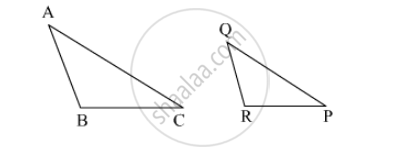∆PQR ~ ∆ABC

∆PQR ~ ∆CAB

∆CBA ~ ∆PQR

∆BCA ~ ∆PQR

Concept: Similarity of Triangles
Chapter:  Similarity
1.A.ii

In a right angled triangle, if sum of the squares of the sides making right angle is 169 then what is the length of the hypotenuse?

(A) 15 (B) 13 (C) 5 (D) 12

15

13

12

Concept: Apollonius Theorem
Chapter:  Pythagoras Theorem
1.A.iii

In a right angled triangle, if sum of the squares of the sides making right angle is 169 then what is the length of the hypotenuse?

(A) 15 (B) 13 (C) 5 (D) 12

15

13

12

Concept: Apollonius Theorem
Chapter:  Pythagoras Theorem
1.A.iv

Choose the correct alternative answer for each of the following questions

(7) Find the volume of a cube of side 0.01 cm.

(A) 1 cm3

B) 0.001 cm

(C) 0.0001 cm3

(D) 0.000001 cm3

Concept: Problems Based on Areas and Perimeter Or Circumference of Circle, Sector and Segment of a Circle
Chapter:  Mensuration
1.B
1.B.i

Find the distance between the following pair of point.

$W\left( \frac{- 7}{2} , 4 \right), X\left( 11, 4 \right)$

Concept: Distance Formula
Chapter:  Co-ordinate Geometry
1.B.ii

Prove that:
$\frac{\sin^2 \theta}{\cos\theta} + \cos\theta = \sec\theta$

Concept: Application of Trigonometry
Chapter:  Trigonometry
1.B.iii

∆PQR ~ ∆LTR. In ∆PQR, PQ = 4.2 cm, QR = 5.4 cm, PR = 4.8 cm. Construct ∆PQR and ∆LTR, such that $\frac{PQ}{LT} = \frac{3}{4} .$

Concept: Division of a Line Segment
Chapter:  Geometric Constructions
1.B.iv

Seg RM and seg RN are tangent segments of a circle with centre O. Prove that seg OR bisects ∠MRN as well as ∠MON.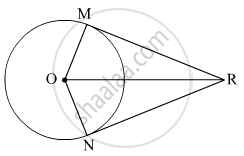Concept: Tangent Segment Theorem
Chapter:  Circle
2
2.A | Solve any 2 of the following
2.A.i

In the given figure, ∠QPR = 90°, seg PM ⊥ seg QR and Q–M–R, PM = 10, QM = 8, find QR.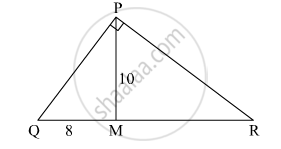Concept: Application of Pythagoras Theorem in Acute Angle and Obtuse Angle
Chapter:  Similarity  Pythagoras Theorem
2.A.ii

The dimensions of a cuboid are 44 cm, 21 cm, 12 cm. It is melted and a cone of height 24 cm is made. Find the radius of its base.

Concept: Surface Area of a Combination of Solids
Chapter:  Mensuration
2.A.iii

Prove that:
$\sec^4 \theta - \cos^4 \theta = 1 - 2 \cos^2 \theta$

Concept: Application of Trigonometry
Chapter:  Trigonometry
2.B | Solve any 4 of the following
2.B.i

In adjoining figure PQ ⊥ BC, AD⊥ BC then find following ratios.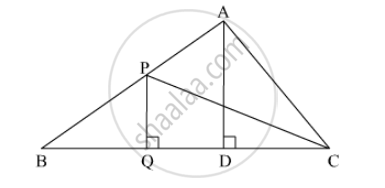(i) $\frac{A\left( ∆ PQB \right)}{A\left( ∆ PBC \right)}$

(ii) $\frac{A\left( ∆ PBC \right)}{A\left( ∆ ABC \right)}$

(iii) $\frac{A\left( ∆ ABC \right)}{A\left( ∆ ADC \right)}$

(iv) $\frac{A\left( ∆ ADC \right)}{A\left( ∆ PQC \right)}$

Concept: Similar Triangles
Chapter:  Similarity
2.B.ii

In the given figure, M is the midpoint of QR. ∠PRQ = 90°. Prove that, PQ= 4PM– 3PR2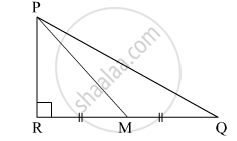Concept: Pythagoras Theorem
Chapter:  Pythagoras Theorem
2.B.iii

While landing at an airport, a pilot made an angle of depression of 20°. Average speed of the plane was 200 km/hr. The plane reached the ground after 54 seconds. Find the height at which the plane was when it started landing. (sin 20° = 0.342)

Concept: Heights and Distances
Chapter:  Trigonometry
2.B.iv

From the top of a lighthouse, an observer looking at a ship makes angle of depression of 60°. If the height of the lighthouse is 90 metre, then find how far the ship is from the lighthouse.

$\left( \sqrt{3} = 1 . 73 \right)$
Concept: Heights and Distances
Chapter:  Trigonometry
2.B.v

Solve the following example.

Find the height of an equilateral triangle having side 2a.

Concept: Apollonius Theorem
Chapter:  Pythagoras Theorem
3
3.A | Solve any 1 of the following
3.A.i

In ∆PQR, PM = 15, PQ = 25 PR = 20, NR = 8. State whether line NM is parallel to side RQ. Give reason.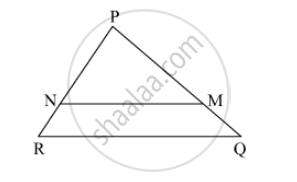Concept: Property of three parallel lines and their transversals
Chapter:  Similarity
3.A.ii

In the adjoining figure, O is the centre of the circle. From point R, seg RM and seg RN are tangent segments touching the circle at M and N. If (OR) = 10 cm and radius of the circle = 5 cm, then
(1) What is the length of each tangent segment ?
(2) What is the measure of ∠MRO ?
(3) What is the measure of ∠ MRN ?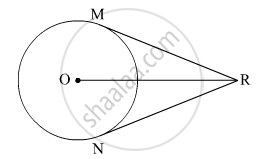Concept: Tangent Segment Theorem
Chapter:  Circle
3.B | Solve any 2 of the following
3.B.i

Draw a circle with radius 3.4 cm. Draw a chord MN of length 5.7 cm in it. construct tangents at point M and N to the circle.

Concept: Construction of Tangents to a Circle
Chapter:  Geometric Constructions
3.B.ii

Find the co-ordinates of the points of trisection of the line segment AB with A(2, 7) and B(–4, –8).

Concept: Division of a Line Segment
Chapter:  Co-ordinate Geometry
3.B.iii

In the given figure, the circles with centres P and Q touch each other at R. A line passing through R meets the circles at A and B respectively. Prove that – (1) seg AP || seg BQ,
(2) ∆APR ~ ∆RQB, and
(3) Find ∠ RQB if ∠ PAR = 35°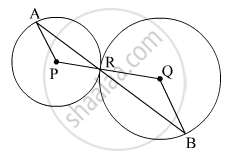Concept: Touching Circles
Chapter:  Circle
3.B.iv

In the given figure shows a toy. Its lower part is a hemisphere and the upper part is a cone. Find the volume and surface area of the toy from the measures shown in the figure ($\pi = 3 . 14$)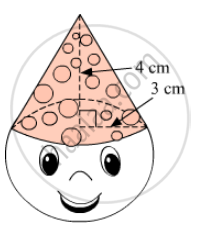Concept: Surface Area of a Combination of Solids
Chapter:  Mensuration
4 | Solve any 2 of the following
4.A

In ΔABC, D and E are points on the sides AB and AC respectively such that DE || BC

If AD = 8x − 7, DB = 5x − 3, AE = 4x − 3 and EC = (3x − 1), find the value of x.

Concept: Basic Proportionality Theorem Or Thales Theorem
Chapter:  Similarity
4.B

Prove that the tangents drawn at the ends of a diameter of a circle are parallel.

Concept: Number of Tangents from a Point on a Circle
Chapter:  Circle
4.C

A solid is in the form of a right circular cylinder, with a hemisphere at one end and a cone at the other end. The radius of the common base is 3.5 cm and the heights of the cylindrical and conical portions are 10 cm. and 6 cm, respectively. Find the total surface area of the solid. (Use n =22/7)

Concept: Surface Area of a Combination of Solids
Chapter:  Mensuration
5 | Solve any 1 of the following
5.A

Draw a circle of radius 3.4 cm and centre E. Take a point F on the circle. Take another point A such that E-F-A and FA = 4.1 cm. Draw tangents to the circle from point A.

Concept: Construction of Tangents to a Circle
Chapter:  Geometric Constructions
5.B

If A (–14, –10), B(6, –2) is given, find the coordinates of the points which divide segment AB into four equal parts.

Concept: Division of a Line Segment
Chapter:  Co-ordinate Geometry

#### Request Question Paper

If you dont find a question paper, kindly write to us

View All Requests

#### Submit Question Paper

Help us maintain new question papers on Shaalaa.com, so we can continue to help students

only jpg, png and pdf files

## Maharashtra State Board previous year question papers 10th Geometry with solutions 2019 - 2020

Maharashtra State Board 10th Geometry question paper solution is key to score more marks in final exams. Students who have used our past year paper solution have significantly improved in speed and boosted their confidence to solve any question in the examination. Our Maharashtra State Board 10th Geometry question paper 2020 serve as a catalyst to prepare for your Geometry board examination.
Previous year Question paper for Maharashtra State Board 10th Geometry-2020 is solved by experts. Solved question papers gives you the chance to check yourself after your mock test.
By referring the question paper Solutions for Geometry, you can scale your preparation level and work on your weak areas. It will also help the candidates in developing the time-management skills. Practice makes perfect, and there is no better way to practice than to attempt previous year question paper solutions of Maharashtra State Board 10th.

How Maharashtra State Board 10th Question Paper solutions Help Students ?
• Question paper solutions for Geometry will helps students to prepare for exam.
• Question paper with answer will boost students confidence in exam time and also give you an idea About the important questions and topics to be prepared for the board exam.
• For finding solution of question papers no need to refer so multiple sources like textbook or guides.
S